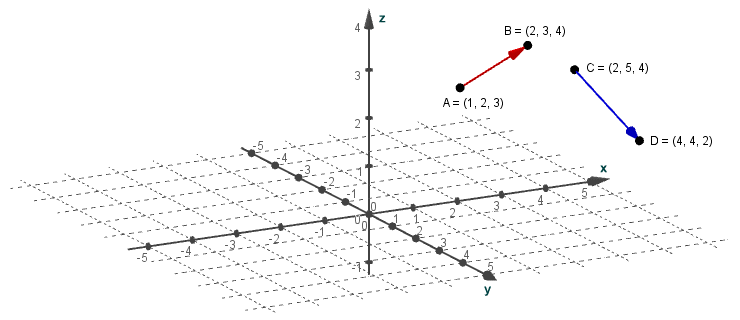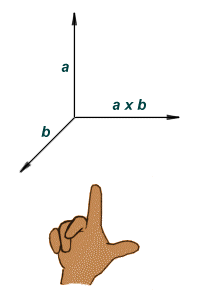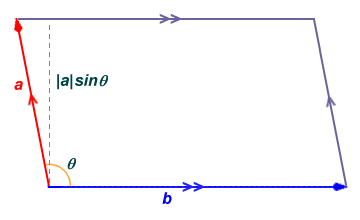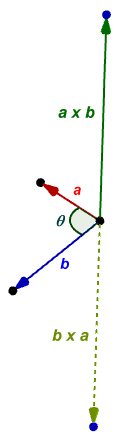# The Cross Product of Two Vectors

There are situations in the study of mathematics, physics or engineering in which we are required to compute the cross product of two vectors. It can be used in mechanics, for example, to find the torque applied by a force, or in the field of computer graphics to calculate the surface normal for a polygon (i.e. the vector that is perpendicular to the surface of the polygon). The cross product is the result of multiplying the vectors together, and will result in a third vector that is perpendicular (i.e. at right angles, or orthogonal) to both of the original vectors. The cross product therefore has no meaning in a two-dimensional environment. In fact, it is only meaningful in a three-dimensional or a seven-dimensional environment (we will limit ourselves to a discussion of the cross product in a three-dimensional environment). Consider the illustration below, which shows two separate vectors in a three-dimensional space.Two vectors in a three-dimensional space

The three-dimensional vectors AB and CD have fixed points of origin (i.e. they are effectively position vectors) and there is no single plane in which they both lie. We can of course move one of the vectors so that the two vectors are tail to tail, in which case there will exist one (and only one) plane common to both vectors. We will move vector AB so that its tail is coincident with that of vector CD. The illustration below shows the two vectors again, this time with a common point of origin. The grid (which lies in the x-y plane) and the point labels have been removed for clarity.The vectors are now tail to tail

What we are really interested in here are the x, y and z components of the vectors, rather than the x, y and z coordinates of their end points. Finding the x, y and z component values is a simple task if we know the x, y and z coordinates of the start and end points for each vector All we need to do to find the x component of a vector, for example, is to subtract the x coordinate of its start point from that of its end point. Once we have the component values for each vector, we can we can simply treat them as free vectors that share a common point of origin and thus lie in the same plane. In the following graphic, we show only the component values, and have removed the x, y and z axes altogether.The component values for vectors a and b

We said above that the cross product of two vectors is a new vector that is perpendicular to both of the original vectors, but that leaves us with a question. There are two directions that are perpendicular to both vectors. Which one is the right direction? The answer to that usually depends on the orientation of the coordinate system being used. Let's assume for the purposes of this discussion that we are dealing with a right-handed coordinate system (other possibilities do of course exist, but we will try and keep things relatively simple). The right-hand rule (illustrated below) can then be applied to determine the direction in which the cross product vector points. Note that the cross product of vectors a and b is written as a x b.Applying the right-hand rule

As you can see from the illustration, if the first and second fingers of the right hand are more or less at right-angles to one another, with the thumb more or less at right-angles to both fingers, then the first finger points roughly in the direction of vector a, the second finger in the direction of vector b, and the thumb in the direction of a × b (i.e. the cross product vector). Note that the angle between vectors a and b can be any angle between zero and one hundred and eighty degrees. Below is a graphic showing vectors a and b, together with the cross product vector, which we have labelled vector c. Note that, because we are dealing with a three-dimensional environment, this is just one possible viewpoint. Keep in mind, however, that vector c is at right-angles to both vector a and vector b.Vectors a and b with their cross product, vector c

The question you should probably be asking yourself at this point is how did we arrive at the x, y and z components for vector c shown in the illustration? Well, without going into lengthy explanations, we find the x, y and z components of vector c (i.e. the cross product vector) by using the x, y and z components of vectors a and b as follows:

cx  =  aybz - azby  =  (1)(-2) - (1)(-1)  =  -1

cy  =  azbx - axbz  =  (1)(2) - (1)(-2)  =  4

cz  =  axby - aybx  =  (1)(-1) - (1)(2)  =  -3

Note that since vector c is perpendicular to the plane in which vectors a and b both lie, it follows that if vectors a and b both lie in the x-y plane, their z component will be zero. Consequently, the x and y components of vector c will also be zero (i.e. vector c will be parallel to the z-axis). Note also that if either vector a or b is the zero vector, or if vectors a and b are parallel, their cross product will also be the zero vector. As long as vectors a and b do not lie in a plane defined by any two out of the three axes (x, y and z), then all of the components of vector c will be non-zero.

We can also express the cross product of two vectors in matrix form. We do this by first creating a three-by-three matrix that contains the x, y and z components of vectors a and b. The first row of the matrix must consist of the orthonormal vectors x, y and z. An orthonormal vector is a unit vector (i.e. it has a magnitude of one) which has only one non-zero component. To illustrate what this means, the orthonormal vectors x, y and z can be represented using the following column matrices:

 x  = 1 0 0
 y  = 0 1 0
 z  = 0 0 1

The three-by-three matrix is presented below. Vectors x, y and z are essentially used as a kind of placeholder to represent the value one in each column of the first row of the matrix. In some representations, you may see different characters used to represent the orthonormal vectors (e.g. i, j and k), and the characters may appear with a caret ("^") above them to signify the fact that they are orthonormal vectors. The second and third rows contain the x, y and z component values of vectors a and b respectively.

 x y z ax ay az bx by bz

The cross product vector is obtained by finding the determinant of this matrix. If you are unfamiliar with matrices, you might want to look at the page on matrices in the Algebra section to see how the determinant of a three-by-three matrix is found. Below is the actual calculation for finding the determinant of the above matrix (i.e. the cross product of vectors a and b). Note that the determinant of a matrix is written in the same format as the original matrix, but instead of brackets, the columns are enclosed within vertical bars.

 c  =  a × b  = x y z =  x ay az -  y ax az +  z ax ay ax ay az by bz bx bz bx by bx by bz

Substituting actual values, we get:

 c  =  a × b  = x y z =  x 1 1 -  y 1 1 +  z 1 1 1 1 1 -1 -2 2 -2 2 -1 2 -1 -2

The calculation then becomes:

c  =  a × b  =  x ((1)(-2) - (-1)(1)) - y ((1)(-2) - (2)(1)) + z ((1)(-1) - (2)(1))

c  =  -x + 4y - 3z

This gives us the x, y and z vector components for the cross product vector directly:

c  =  (-1, 4, -3)

Note that the magnitude of vector c (the cross product vector) is actually the same as the area of a parallelogram for which vectors a and b provide adjacent sides, as shown below.Vectors a and b can provide adjacent sides of a parallelogram

The area of a parallelogram is obtained by multiplying the length of the base of the parallelogram by its height. In the above example, we have assumed that vector b will be the base of the parallelogram. The height of the parallelogram is obtained by multiplying the length of either of the sides adjacent to the base by the sine of any one of its internal angles. In the above example, we have used vector a and the sine of angle θ. The area of the parallelogram constructed using vectors a and b, and consequently the magnitude of vector c, is given by:

|c|  =  |a × b|  =  |a||b| sin (θ )

Of course, we need to obtain values for angle θ (the angle between vectors a and b), and the magnitudes of vectors a and b, before we can perform this calculation. Once you have these values, you don't need to understand how the trigonometric sine function works in order to obtain a valid answer, but you do need to know how to use the sine button on your calculator correctly.

There is one other thing to be aware of before we move on which is that, unlike the dot product of two vectors, the cross product is not commutative. In other words, a × b produces a different result from b × a. In fact, the cross product b × a has exactly the same magnitude as the cross product a × b, but points in the opposite direction (b × a = -(a × b)).The cross product b × a points in the opposite direction to a × b

At the moment, we are dealing with a few unknowns. We don't know the magnitudes of vectors a and b, we don't have a value for angle θ (which will be the minimum angle through which one of the vectors must be rotated in order to point in the same direction as the other vector), and we don't know the x, y and z components of the unit vector n. How do we find these missing values? We'll start by finding the magnitudes (i.e. lengths) of vectors a and b, which is relatively straightforward:

|a|  =  √ax2 + ay2 + az2  =  √1 + 1 + 1  =  √3  =  1.732

|b|  =  √bx2 + by2 + bz2  =  √4 + 1 + 4  =  √9  =  3

To find angle θ, we can use the dot product of vectors a and b (if you are unfamiliar with the dot product of two vectors, have a look at the relevant page in this section). We can find the dot product of vectors a and b using the information we already have as follows:

a · b  =  axbx + ayby + azbz  =  ((1)(2)) + ((1)(-1) + (1)(-2))  =  2 - 1 - 2  =  -1

Since the cosine equation for the dot product of a and b is:

a · b  =  |a||b| cos (θ )

we can rearrange this equation to find θ:

 θ  =  cos-1 a · b |a||b|

Substituting actual values we get:

 θ  =  cos-1 -1 =  cos-1 (-0.192)  =  101.10° 1.732 × 3

Note that the sign of the dot product is important. If you get that wrong, you will get the supplement of angle θ (i.e. the difference between θ and one hundred and eighty degrees) rather than θ.

It only remains to find the x, y and z components of unit vector n. Bearing in mind that we already have the x, y, and z components of vector c, this is fairly straightforward. The unit vector by definition has a length of one unit, so the total length of vector c would tell us the scalar value by which unit vector n would have to be multiplied in order to get vector c. We can therefore divide the x, y and z components of vector c by its length to get the x, y and z components of unit vector n. To find the length of vector c, we can use the following calculation:

|c|  =  √cx2 + cy2 + cz2  =  √1 + 16 + 9  =  √26  =  5.099

The components of unit vector n are then found as follows:

nx  =  -1 ÷ 5.099  =  -0.196

ny  =  4 ÷ 5.099  =  0.784

nz  =  -3 ÷ 5.099  =  -0.588

We already have an alternative equation for the magnitude of vector c (see above):

|c|  =  |a × b|  =  |a||b| sin (θ )

Since the direction of vector c is given by the unit vector n, we can obtain an alternative equation for vector c (i.e. the cross product vector) simply by including the term n:

c  =  a × b  =  |a||b| sin (θ ) n

If we now substitute actual values into our equation, we should be able to obtain the same set of vector components for vector c as we got from our original calculation using the x, y and z components of vectors a and b:

c  =  1.732 × 3 × sin (78.93°) n  =  5.099 n

c  =  5.099 × (-0.196, 0.784, -0.588)  =  (-0.999, 3.998, -2.998)

So, the cross product of vectors a and b in row vector form is (-0.999, 3.998, -1.998). Rounding these numbers up, we get (-1, 4, -2). These are the vector components cx, cy and cz that we arrived at previously. Note that because n is the unit vector (i.e. it has a magnitude of one unit), its sole purpose in the equation is to define the direction of the cross product vector. This should be whichever of the two directions perpendicular to both vector a and vector b was selected by using the right-hand rule. Obviously, in order to use this equation to obtain vector c, we would need to know the magnitudes of vectors a and b, the value of angle θ, and the values of the x, y and z components of vector n. The method actually used to find the cross product of two vectors will therefore very much depend upon what information we are given to start with.